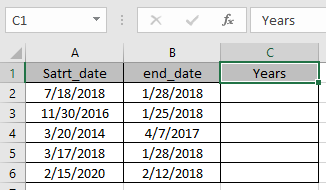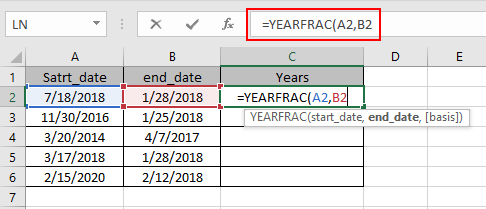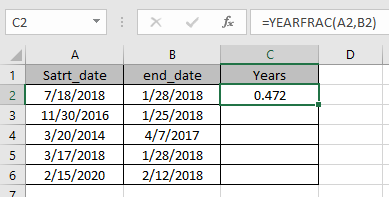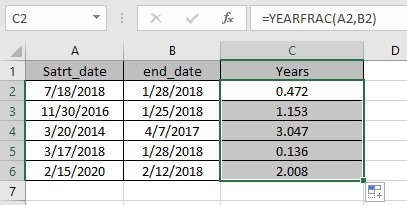# How to use the YEARFRAC Function in Excel

YEARFRAC function calculates the fraction of year between the start_date & end_date. A period of six month is half a year which is 0.5 years. So to view the results in the year format use the YEARFRAC function.

The YEARFRAC function is a built-in financial function which returns the fraction of year between the start_date & end_date.

Syntax:

=YEARFRAC ( start_date, end_date, [basis])

Start_date : A valid date which represents the start date
end_date : A valid date which represents the end date
basis : [optional] Day count basis. Default is 0.

 Basis Day count basis 0 US (NASD) 30 / 360 1 actual / actual 2 actual / 360 3 actual / 365 4 European 30 / 360

Let’s understand this function using it in an example.Here we have values to calculate the YEARFRAC value between the two given dates.

Use the formula:

=YEARFRAC ( A2, B2 )

A2 : start date given as cell reference.
B2 : end date given as cell reference.
B5 : 0 (default) specifies Day count basis method choose US (NASD) 30 / 360 .[optional]Here all the arguments to the function is given as cell reference.

Note: Dates in the formula must be entered using cell reference or DATE function. Excel date format creates #VALUE! error while computing results. Use the cell reference or DATE function in excel while taking date as input to the function

Now Press Enter to get the YEARFRAC valueThe result specifies the year gap between the two dates.
Now copy the formula to other cells using the Ctrl + D shortcut key.As you can see here, the function works fine. Now see the below notes to remember some observational mistakes

Note:

1. Excel stores dates as serial number and is used in calculation by the function. So it’s recommended to use dates as cell reference instead of giving direct argument to the function.
2. The function returns the #VALUE! Error if the input start_date & end _date are not a valid date.
3. The function returns the #NUM! Error if the value of argument basis is either < 0 or  > 4.

Hope you understood how to use YEARFRAC function and referring cell in Excel. Explore more articles on Excel function here. Please feel free to state your query or feedback for the above article.

Related Articles:

How to Use SUBTOTAL Function in Excel

How to use the Excel LOG10 function

How to use the IMEXP Function in Excel

How to use the IMCONJUGATE Function in Excel

How to use the IMARGUMENT Function in Excel

Popular Articles

Edit a dropdown list

If with conditional formatting

If with wildcards

Vlookup by date

Terms and Conditions of use

The applications/code on this site are distributed as is and without warranties or liability. In no event shall the owner of the copyrights, or the authors of the applications/code be liable for any loss of profit, any problems or any damage resulting from the use or evaluation of the applications/code.